Name:    1MD3

Multiple Choice
Identify the choice that best completes the statement or answers the question.

1.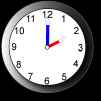What time does this clock say?
 a. 2:00 c. 12:00 b. 1:00 d. 11:00

2.

Which digital clock below matches this analog clock?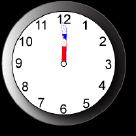a.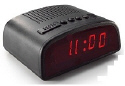c.b.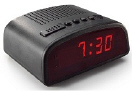d.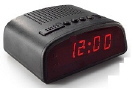3.

Which analog clock below matches this digital clock?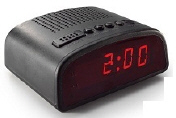a.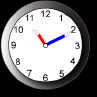c.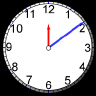b.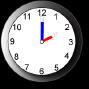d.4.

Which digital clock below shows the same time as this analog clock?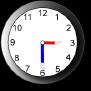a.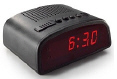c.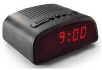b.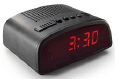d.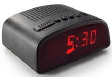5.

What time does this clock say?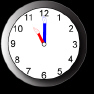a. 6:00 c. 12:00 b. 11:00 d. 12:30

6.

What time does this clock say?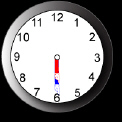a. 6:15 c. 6:30 b. 7:00 d. 6:00

7.

What time does this clock say?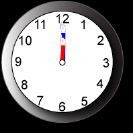a. 6:00 c. 12:30 b. 12:00 d. 12:15

8.

Which digital clock below matches this analog clock?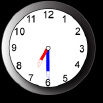a.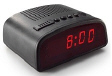c.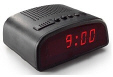b.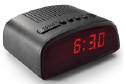d.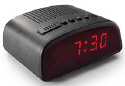Completion
Complete each statement.

9.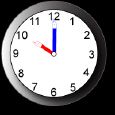What time does this clock say?

10.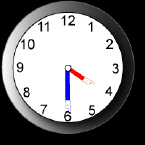What time does this clock say?

11.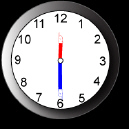What time does this clock say?